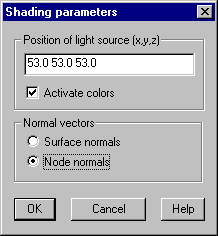For displaying shaded structures, the position of the light source and the kind of normal vectors to be used must be set in the following dialog:Position of light source (x, y, z)

Give the position of the light source in the input field.

Activate colors: With this option set the elements are plotted with associated colors. Otherwise grayscales are used.

Normal vectors

For calculation of light intensities normal vectors of the surfaces are needed. Therefore it’s important that all surfaces use correct node ordering (see command Check

Surface normals: Only the normal vector of element surface is used for calculation of light intensity.

Node normals: On each node a normal vector is calculated as a mean value of all adjacent elements, this gives a smooth transition of intensities among elements.